Disclaimer: This is an example of a student written essay.

Any opinions, findings, conclusions or recommendations expressed in this material are those of the authors and do not necessarily reflect the views of UKEssays.com.

# Skew Detection of Devanagari Script Using Pixels

 ✅ Paper Type: Free Essay ✅ Subject: Computer Science ✅ Wordcount: 3682 words ✅ Published: 4th Apr 2018

Skew Detection of Devanagari Script Using Pixels of Axes-Parallel Rectangle and Linear Regression

• Trupti A. Jundale

Abstract—Skew detection and correction of handwritten data is one of the difficult tasks in pattern recognition area. Here we illustrate the method for skew detection and correction of Devanagari handwritten script. The proposed approach works for single skew. The input images for this research are collected from various writers and contain single/uniform skew words/lines. The proposed approach uses tangential pixels of axes parallel rectangle and linear regression method to calculate the skew of word/line. Finally rotation transformation is used for correction of skew of word/line which is calculated by linear regression. This technique achieves 89% accuracy to correct skew of word and achieves 93% accuracy to correct skew of line for handwritten Devanagari script.

Index Terms—Preprocessing, Axes-parallel rectangle, Linear Regression, Skew detection, Skew correction

## I. Introduction

The frequency of digital document extends to develop at a brisk rate in spite of the usage of paper based documents. As a result, the renovation of paper documents to its electronic version and its consequent image processing and understanding have been converted into a vital application area in computer vision and pattern recognition researches. With recent emergence and widespread application of multimedia technologies, there is an increasing demand to create a paperless environment, hence, document image processing in general and Optical Character Recognition (OCR) in particular is playing an important role in transformation of the traditional paper based environment to truly paperless electronic environment.

If you need assistance with writing your essay, our professional essay writing service is here to help!

Devanagari is one of the mainly used and espouses writing system in the world. The national/official language of India (Hindi) and Nepal (Nepali) uses Devanagari Script. Many other languages like Marathi (state language of Maharashtra), Sanskrit, Kashmiri, Bhojpuri, Maithili, Bodo, Dogri etc. comes under Devanagari Script. As India’s national language uses Devanagari script, lot of official data is in written format before the era of digitization. So in the today’s world of digitization, it is needed to keep record of handwritten/printed data in digital form. To make this, Optical Character Recognition (OCR) system is carried out. The detection and correction of skew is one of the essential steps in any character recognition or document processing system. Because of the writing genre of the Devanagari script, it is difficult to detect skew as compared to any other script. The writing style of every person may vary so there is presence of multiple skew in data. Skew is the angle which diverges from x-axis. The successful skew detection and correction turns next process like analysis of character or OCR to be accurate. The document may contain three type of skew: single/uniform skew, multiple skew and non-uniform skew. Single/uniform skew is, when all text lines in a document have same orientation. Multiple skew is, when some text lines have different orientation than other and non-uniform skew is, when orientation changes within a line. There is lot of research available for skew detection of scanned document image but less work is available for skew detection of text/word.

## II. Devanagari script

One of the main parts of Brahmic family is a Devanagari Script, which is belonging from Indo-Aryan languages. It is written from left to right. Unlike Latin script, concept of upper/lower case is absent in Devanagari script. It consists of 33 consonants and 14 vowels. Generally every word written in most of the Devanagari Script will have a header line on group of characters, called as ‘Shirorekha’ and this is considered as one word . Vowels that can be written as separate characters or by using diacritic marks on below, upper, before or after consonants are called modifiers. In Devanagari script, two or three consonants can be written as a single character, which is known as compound character. Fig.1 shows different features of Devanagari script.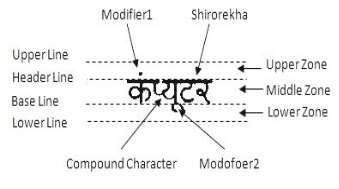Fig. 1. Devanagari Script Word

The main characters of word are written in middle zone. Upper zone and lower zone are for modifiers and Shirorekha is drawn at header line. In Fig.1 two characters are combined to form a new shape of single character known as compound character.

## III. Related Work

In the literature, algorithms that estimate the angle at which a text/document image is rotated are surveyed. The broad classes of technique are identified, which include methods that calculate skew from Hough transform, horizontal projection profile, Fourier transform, nearest-neighbour or principal component analysis. The basic method used by each class of technique is presented and the contributions of individual algorithms within each class are discussed.

Hough Transform: One of the best feature extraction technique used in digital image processing and computer vision is Hough Transform. It is mainly used for detection of regular curves such as lines, ellipses, circles etc. The simplest case of Hough transform is the linear transform for detecting straight lines. The line in the image space is just a single point in the parameter space.  uses Hough transform method for detection of document skew. A novel skew correction algorithm is proposed focusing on boundary line that optimizes speed and accuracy by using Hough transform to get the skew corrected licences plate images in .

Fourier Transform: In this method first 2-D Fourier transform will be applied to the image plane. Then, coefficients of the power spectrum are calculated and stored in a spectrum. A directional criterion for each angle is then calculated. The angle that maximizes the directional criterion is assumed to give the skew angle of the image.

Projection Profile: Projection Profile can be a horizontal projection profile or vertical projection profile. The horizontal/vertical projection profile is a histogram of the number of black pixels along horizontal/vertical scan lines. In projection profiles, histogram is created at each possible angle and a ‘cost function’ is applied to this histogram. The skew angle is the angle at which this cost function is maximized. Mostly horizontal projection profile method is used for scanned document skew detection.  exploits the unique property of the writing line of Arabic script and is based on connected component analysis and projection profiles. Skew detection of fabric images scheme based on morphological method and projection profile analysis is proposed in .

Nearest Neighbour: In Nearest Neighbour method histogram of the direction angle is computed.  uses a Focused Nearest Neighbour Clustering (FNNC) of interest points and the analysis of paragraphs/lines. Chains with a largest possible number of nearest neighbour pairs are selected and their slopes are computed to give the skew angle of document image.

Other than these techniques, one-step skew and orientation detection method using a well-established geometric text-line model is used in . The advantage of this method is that it combines accurate skew estimation with robust, resolution-independent orientation detection.  proposed a Rectangular Active Contour Model (RAC Model) for content region detection and skew angle calculation by imposing a rectangular shape constraint on the zero-level set in Chain-Vese Model (C-V Model) according to the rectangular feature of content regions in document images. B. V. Dhandra et.al,  uses image dilation and region labelling approach for binary document skew detection. Apart from this, fast and robust skew estimation techniques like a bilinear filtering model which is used to detect edges existing in the document, COG (Centre of Gravity) method are used in the literature.

## IV. Proposed Methodology

This section illustrates the proposed methodology for skew detection and correction. Section A describes pre-processing step. Section B describes extraction of axes parallel rectangle pixels. Skew detection using linear regression is described in C. Section D describes skew correction technique and last section E describes steps of proposed algorithm.

## A. Pre-processing

The input to the system is a word or a line of single/uniform skew of handwritten Devanagari script which is scanned by optical scanner or captured by digital camera. Acquired input is pre-processed for removing noise. Firstly input image is converted into gray scale image and then thresholding is applied over for converting given image into binary image containing only black and white pixels. In this binarized image, white pixels represent background and black pixels represent foreground.

## B. Axes-Parallel Rectangle

This stage calculates the area of axes-parallel rectangle. The angle with the least area of the axes-parallel rectangle represents the skew angle. Outer tangential pixels of an input word/line are used to form an axes-parallel rectangle. Figure 2 shows tangential pixels of skewed one are embedded into an axes-parallel rectangle.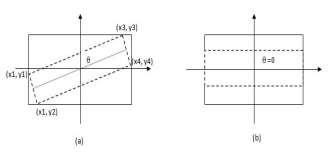Fig. 2 (a) Skewed rectangle fitted in an Axes-parallel rectangle (b) Rectangle with zero skew.

## C. Skew Detection

After getting required pixels using axes-parallel rectangle, linear regression formula is used to detect skew of word/line. Regression analysis can be used to identify the line or curve which provides the best fit through a set of data points. Linear regression analyzes the relation between two variables, X and Y. The variables X and Y are known and the problem is to fit best straight line through X and Y. In general, the goal of linear regression is to find the line that best predicts Y from X. Linear regression does this by finding the line that minimizes the sum of the squares of the vertical distances of the points from line. Linear regression does not test whether the data are linear. It assumes that the data is linear, and finds the slope and intercept that make a straight line best fit the given data. The goal of linear regression is to adjust the values of slope and intercept to find the line that best predicts Y from X.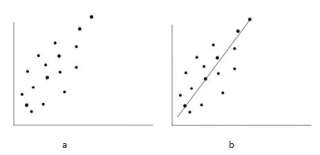Fig. 3 (a) Plot of data without best-fit line (b) Plot of data with best-fit line.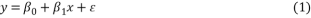This is the simple linear regression model where ï¢0 and ï¢1 are unknown constants and ï¥ is the residual error. To fit the regression line in the equation of the data (x1, y1), (x2, y2),…..,(xn, yn) by finding best match between the line and the data. The best choice of ï¢0+ï¢1 will be chosen to minimize,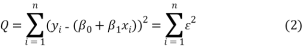This is called the least square fit. The equation (2) implies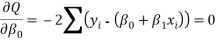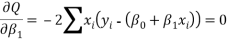ïƒ›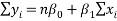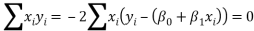After little algebra, get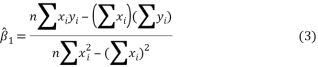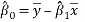where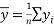and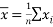(4)

Equation (3) gives slope of the regression line and Equation (4) gives the intercept. The slope of the line is nothing but the skew angle of our word/line. Fig.4 shows the slope and intercept of a best fit line.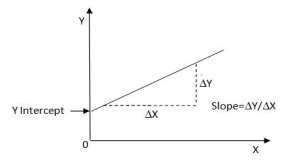Fig. 4 Slope and intercept of a best fit line

After calculating slope using linear regression, skew is calculated using the formula,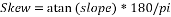This gives the required skew of word.

## D. Skew Correction

After the skew angle of the word/line has been detected, the word/line must be rotated in order to correct this skew. Various methods used for skew correction are direct method, indirect method and contour-oriented method etc. The direct method uses rotation transformation in which corresponding pixels in the input image will be transformed to new location by using equation (1)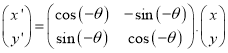(5)

Where (x, y) are the co-ordinates of pixels belonging to the word for which skew has to be detected and (x’, y’) are the co-ordinates of pixels belonging to the word after correction. For a pixel (x’, y’) in the output image, the indirect method finds corresponding pixel in the input image and assigns a value of (x’, y’) to (x, y) using Equation (2).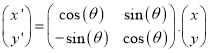(6)

We apply direct method for skew correction which simply rotate calculated skew angle to horizontal angle. The detected angle by linear regression is corrected by applying rotation transformation. The word/line is rotated with θ angle. The word/line is corrected by rotating at positive angle if the skew detection angle is negative and corrected by rotating at negative angle if skew detection angle is positive.

## E. Algorithm

Step 1: Accept the input image which may be word or line.

Step 2: Convert the given input into binary by using thresholding method.

Step 3: Calculate the axes-parallel rectangle of binary image by finding minimum row and minimum column pixels.

Step 4: Apply linear regression, Equation (3), to detect the skew of axes-parallel rectangle, which is the skew of original word/line.

Step 5: Using Equation (6), correct the skew angle of word/line.

## V. Experimental Result

We tested our algorithm for input images of handwritten document for Hindi and Marathi languages. The algorithm is tested on 500 words and 300 lines of Devanagari script. The accuracy rate for skew correction of word is 89% and accuracy rate for uniform skew correction of line is 93%.Mostly the word with single character or small size length does not give accurate result because of the lack of a sufficient number of minima points. Table I shows the sample results of words with skew detection of positive and negative angle and skew correction of all these.

1. Results of word skew
 Original word Axes-parallel rectangle Skew Correction Positive skew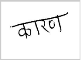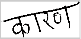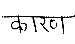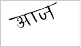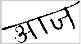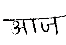Negative skew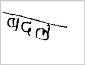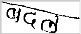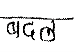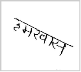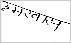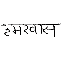Figure 5 shows skew detection and correction of uniform skew line. We tested our algorithm for document with single/uniform skew and for skewed printed document also. For these kinds of input images, algorithm runs successfully.

## VI. Conclusion

We have proposed a methodology for skew detection and correction of word and line of handwritten Devanagari script. The slope of best line fit using linear regression algorithm is used for skew detection and it is corrected by simply rotating word/line by calculated angle. This method is tested on handwritten data of Hindi and Marathi language. The word dataset is collected from various writers for testing purpose which contains 500 words and 300 lines. The proposed approach can be modified for future work to get higher accuracy and for detection of documents contain multiple or non-uniform skew text.

##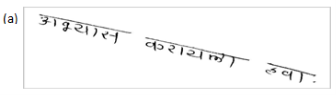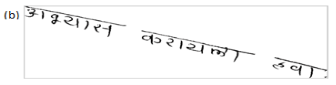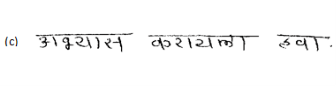Fig. 5 (a) Skewed line (b) Axes-parallel rectangle of skewed line (c) Skew correction of line

## VII. References

1. Deepak Kumar, Dalwinder Singh, “Modified approach of Hough transform for skew detection and correction in documented images”, International Journal of Research in Computer Science, Vol. 2, Issue 3, pp. 37-40, April 2012.
2. Arulmozhi K., Perumal S. A., Priyadarshini C.S.T., Nallaperumal K., “Image refinement using skew angle detection and correction for Indian licences plates”, Computational Intelligence & Computing Research (ICCIC), IEEE, pp. 1-4, Dec. 2012.
3. B.V.Dhandra, H.Mallikarjun, Ravindra Hegadi, V.S.Malemath, “Word-wise Script Identification from Bilingual Documents Based on Morphological Reconstruction”, Visual Information Engineering, IEEE, pp 389-395, 2006.
4. Kleber, Florian, Markus Diem, Robert Sablatnig, “Robust Skew Estimation of Handwritten and Printed Documents Based on Grayvalue Images”, International Conference on Pattern Recognition (ICPR), pp. 3020 – 3025, Aug. 2014.
5. Ahmad Irfan, “A Technique for Skew Detection of Printed Arabic Documents”, Computer Graphics, Imaging and Visualization (CGIV), IEEE, pp. 62-67, Aug. 2013.
6. Trupti A. Jundale, Ravindra S. Hegadi, “Skew Detection and Correction of Devanagari Script Using Hough Transform”, International Conference on Advanced Computing Technologies and Applications, Procedia of Computer Science, Journal of Elsevier, March2015, in press.
7. Liu, Zhoufeng, Jie Huang, Chunlei Li, “Skew detection of fabric images based on edge detection and projection profile analysis”, Foundations of Intelligent Systems, Springer Berlin Heidelberg, Vol. 122, pp 483-488, 2012.
8. H. K. Kwag, S. H. Kim, S. H. Jeony and G. S. Lee, “Efficient skew estimation and correction algorithm for document images”, Image and vision Computing, Vol. 20, pp. 25-35, Jan. 2002.
9. van Beusekom, Joost, Faisal Shafait, and Thomas M. Breuel, “Combined orientation and skew detection using geometric text-line modeling”, International Journal on Document Analysis and Recognition (IJDAR), Vol. 13, Issue 2, pp 79-92, June 2010.
10. Fan, Huijie, Linlin Zhu, and Yandong Tang, “Skew detection in document images based on rectangular active contour”, International Journal on Document Analysis and Recognition (IJDAR), Vol. 13.4, pp 261-269, Dec. 2010.
11. B. V. Dhandra, V. S. Malemath, H. Mallikarjun and R. Hegadi, “Skew detection in binary image documents based on image dilation and region labelling approach” International Conference on Pattern Recognition, IEEE, Vol. 2. pp 954-957, 2006.

View all

## DMCA / Removal Request

If you are the original writer of this essay and no longer wish to have your work published on UKEssays.com then please:

Related Services

Prices from

HK\$1187

Approximate costs for:

• 1000 words
• 7 day deliveryHumanity University

Dedicated to your worth and value as a human being!

Related Lectures